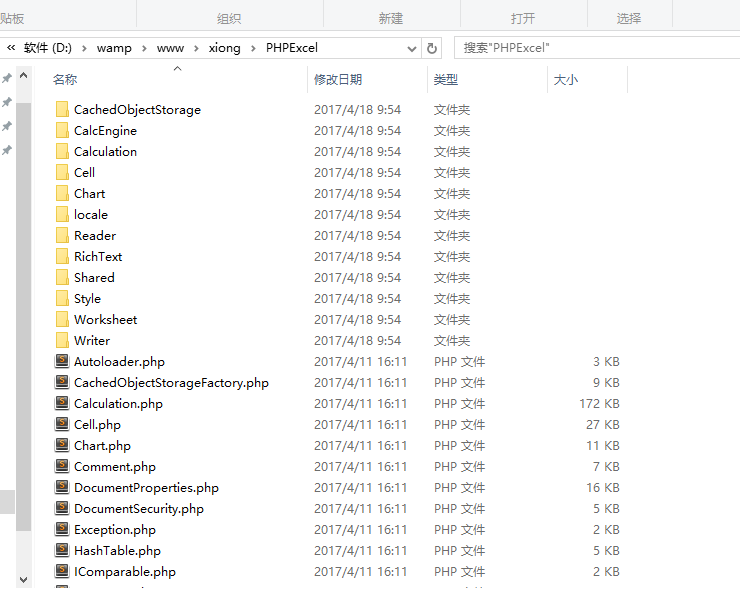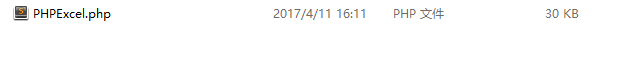# PHP读取Excel文件的方法

PHPExcel开源类库1.读取Excel文件

2.获取最大行号和最大列号

3.通过行数循环里面嵌套列数循环来用特殊符号拼接每个小表格里面的数据得到一个字符串

4.然后使用explode拆分函数将字符串拆分后就得到了一个二维数组（即表格里面的数据）

php代码

`require_once "./PHPExcel.php";\$filePath = "./aa.xlsx";\$PHPReader = new PHPExcel_Reader_Excel2007();if (!\$PHPReader->canRead(\$filePath)) {    \$PHPReader = new PHPExcel_Reader_Excel5();    if (!\$PHPReader->canRead(\$filePath)) {        echo 'no Excel';        exit;    }}\$PHPExcel = \$PHPReader->load(\$filePath);/** * 读取excel文件中的第一个工作表 */\$currentSheet = \$PHPExcel->getSheet(0);/** * 取得最大的列号 */\$allColumn = \$currentSheet->getHighestColumn();/** * 取得一共有多少行 */\$allRow = \$currentSheet->getHighestRow();/** * 从第二行开始输出，因为excel表中第一行为列名 */\$val = '';for (\$currentRow = 1; \$currentRow <= \$allRow; \$currentRow++) {    /**     * 从第A列开始输出     */    for (\$currentColumn = 'A'; \$currentColumn <= \$allColumn; \$currentColumn++) {        \$val .= \$currentSheet->getCellByColumnAndRow(ord(\$currentColumn) - 65, \$currentRow);        /**         * ord()将字符转为十进制数         */        \$val .= "&%|%&";        /**         * 如果输出汉字有乱码，则需将输出内容用iconv函数进行编码转换，如下将GBK编码转为UTF-8编码输出         */                      \$val .= iconv('GBK','UTF-8', \$val);    }    \$val .= "/n";}\$member_info_arr = explode("/n", \$val);unset(\$member_info_arr[count(\$member_info_arr) - 1]);\$present_time = date("Y-m-d H:i:s");if (count(\$member_info_arr) <= 0) {    echo "会员信息文件中无数据，请添加";}unset(\$member_info_arr);foreach (\$member_info_arr as \$key => \$val) {    \$arr[\$key] = explode("&%|%&", \$val);} foreach (\$arr as \$key => \$val) {    unset(\$arr[\$key]);}var_dump(\$arr);`